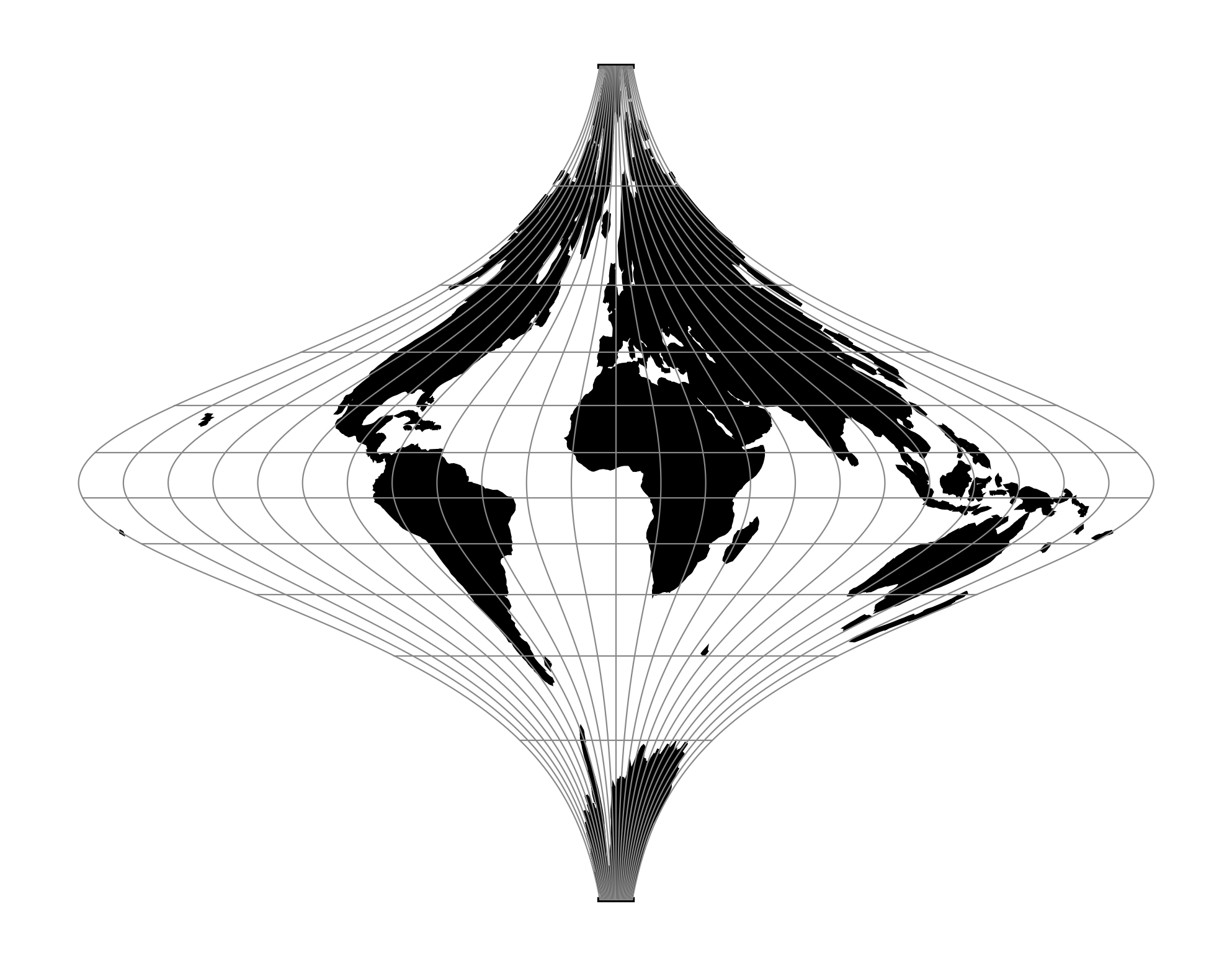# Tobler-Mercator¶

New in version 6.0.0.

Equal area cylindrical projection with the same latitudinal spacing as Mercator projection.

 Classification Cylindrical equal area Available forms Forward and inverse, spherical only Defined area Global, conventionally truncated at about 80 degrees north and south Alias tobmerc Domain 2D Input type Geodetic coordinates Output type Projected coordinatesproj-string: +proj=tobmerc

## Usage¶

The inappropriate use of the Mercator projection has declined but still occasionally occurs. One method of contrasting the Mercator projection is to present an alternative in the form of an equal area projection. The map projection derived here is thus not simply a pretty Christmas tree ornament: it is instead a complement to Mercator’s conformal navigation anamorphose and can be displayed as an alternative. The equations for the new map projection preserve the latitudinal stretching of the Mercator while adjusting the longitudinal spacing. This allows placement of the new map adjacent to that of Mercator. The surface area, while drastically warped, maintains the correct magnitude.

## Parameters¶

Note

All parameters for the projection are optional.

+k_0=<value>

Scale factor. Determines scale factor used in the projection.

Defaults to 1.0.

+lon_0=<value>

Longitude of projection center.

Defaults to 0.0.

+x_0=<value>

False easting.

Defaults to 0.0.

+y_0=<value>

False northing.

Defaults to 0.0.

+R=<value>

Radius of the sphere given in meters. If used in conjunction with +ellps +R takes precedence.

## Mathematical definition¶

The formulas describing the Tobler-Mercator are taken from Waldo Tobler’s article [Tobler2018]

### Spherical form¶

For the spherical form of the projection we introduce the scaling factor:

$k_0 = \cos^2 \phi_{ts}$

#### Forward projection¶

$x = k_0 \lambda$
$y = k_0 \ln \left[ \tan \left(\frac{\pi}{4} + \frac{\phi}{2} \right) \right]$

#### Inverse projection¶

$\lambda = \frac{x}{k_0}$
$\phi = \frac{\pi}{2} - 2 \arctan \left[ e^{-y/k_0} \right]$